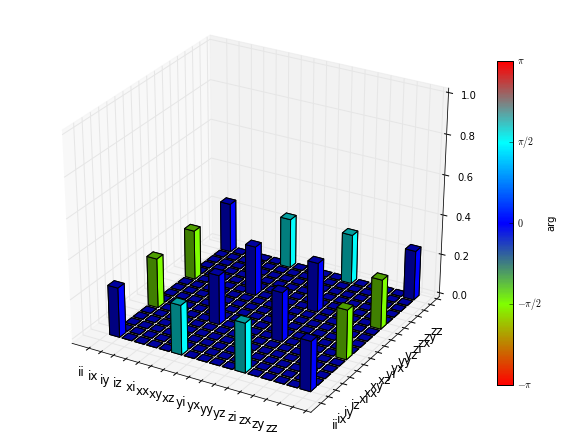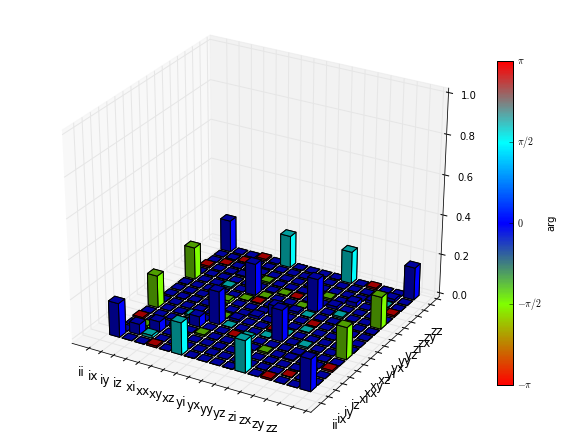# Lecture 7 - Two-qubit iSWAP gate and process tomography¶

Author: J. R. Johansson ([email protected]), http://dml.riken.jp/~rob/

The latest version of this IPython notebook lecture is available at http://github.com/jrjohansson/qutip-lectures.

The other notebooks in this lecture series are indexed at http://jrjohansson.github.com.

In :
%matplotlib inline
import matplotlib.pyplot as plt
import numpy as np

In :
from qutip import *


### Introduction¶

Let's consider a simple protocol for implementing the two-qubit iSWAP gate: During a time $T=\pi/4g$, the qubits are subject to the coupling Hamiltonian

$\displaystyle H = g \left(\sigma_x\otimes\sigma_x + \sigma_y\otimes\sigma_y\right)$

where $g$ is the coupling strength. Under ideal conditions this coupling realizes the $i$-SWAP gate between the two qubit states.

Here we will solve for the dynamics of the two qubits subject to this Hamiltonian, and look at the deterioating effects of adding decoherence. We will use process tomography to visualize the gate.

### Parameters¶

In :
g = 1.0 * 2 * pi # coupling strength
g1 = 0.75        # relaxation rate
g2 = 0.25        # dephasing rate
n_th = 1.5       # bath temperature

T = pi/(4*g)


### Hamiltonian and initial state¶

In :
H = g * (tensor(sigmax(), sigmax()) + tensor(sigmay(), sigmay()))
psi0 = tensor(basis(2,1), basis(2,0))


### Collapse operators¶

Define a list of collapse operators that describe the coupling of the qubits to their environment (assumed to be uncorrelated)

In :
c_ops = []

# qubit 1 collapse operators
sm1 = tensor(sigmam(), qeye(2))
sz1 = tensor(sigmaz(), qeye(2))
c_ops.append(sqrt(g1 * (1+n_th)) * sm1)
c_ops.append(sqrt(g1 * n_th) * sm1.dag())
c_ops.append(sqrt(g2) * sz1)

# qubit 2 collapse operators
sm2 = tensor(qeye(2), sigmam())
sz2 = tensor(qeye(2), sigmaz())
c_ops.append(sqrt(g1 * (1+n_th)) * sm2)
c_ops.append(sqrt(g1 * n_th) * sm2.dag())
c_ops.append(sqrt(g2) * sz2)


### Process tomography basis¶

In :
op_basis = [[qeye(2), sigmax(), sigmay(), sigmaz()]] * 2
op_label = [["i", "x", "y", "z"]] * 2


### Ideal evolution (nondissipative, perfect timing, etc)¶

In :
# calculate the propagator for the ideal gate
U_psi = (-1j * H * T).expm()

In :
# propagator in superoperator form
U_ideal = spre(U_psi) * spost(U_psi.dag())

In :
# calculate the process tomography chi matrix from the superoperator propagator
chi = qpt(U_ideal, op_basis)

In :
fig = plt.figure(figsize=(8,6))
fig = qpt_plot_combined(chi, op_label, fig=fig)

/usr/lib/python3/dist-packages/mpl_toolkits/mplot3d/axes3d.py:1673: RuntimeWarning: invalid value encountered in true_divide
for n in normals])
/usr/lib/python3/dist-packages/matplotlib/colors.py:395: RuntimeWarning: invalid value encountered in greater
if (c.ravel() > 1).any() or (c.ravel() < 0).any():
/usr/lib/python3/dist-packages/matplotlib/colors.py:395: RuntimeWarning: invalid value encountered in less
if (c.ravel() > 1).any() or (c.ravel() < 0).any():### Dissipative evolution¶

In :
# dissipative gate propagator
U_diss = propagator(H, T, c_ops)

In :
# calculate the process tomography chi matrix for the dissipative propagator
chi = qpt(U_diss, op_basis)

In :
fig = plt.figure(figsize=(8,6))
fig = qpt_plot_combined(chi, op_label, fig=fig)### Software versions:¶

In :
from qutip.ipynbtools import version_table

version_table()

Out:
SoftwareVersion
IPython2.0.0
Numpy1.8.1
matplotlib1.3.1
Cython0.20.1post0
SciPy0.13.3
OSposix [linux]
Python3.4.1 (default, Jun 9 2014, 17:34:49) [GCC 4.8.3]
QuTiP3.0.0.dev-5a88aa8
Thu Jun 26 15:03:32 2014 JST